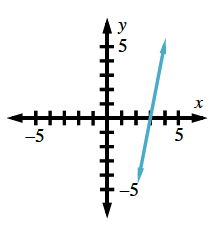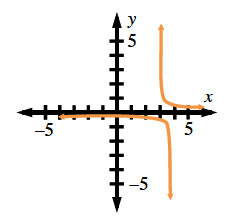### Home > PC > Chapter 5 > Lesson 5.2.3 > Problem5-79

5-79.

Use a graph or a table to compute the following limits.

1. $\lim\limits _ { x \rightarrow 3 } ( x ^ { 2 } - 9 )$1. $\lim\limits_ { x \rightarrow 3 ^ { + } } \frac { 1 } { x ^ { 2 } - 9 }$1. $\lim\limits_ { x \rightarrow 3 ^ { - } } \frac { 1 } { x ^ { 2 } - 9 }$

1. $\lim\limits_ { x \rightarrow 3 } \frac { 1 } { x ^ { 2 } - 9 }$

Are the left and the right limits the same?